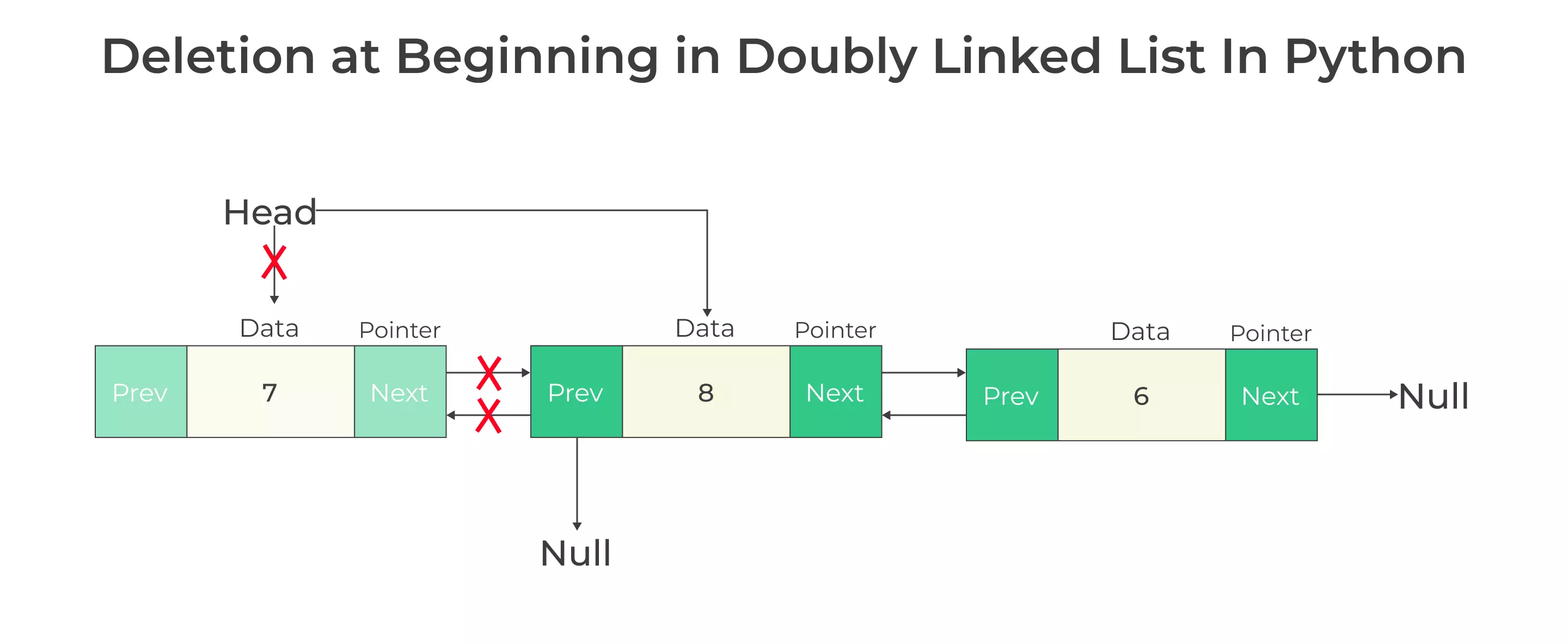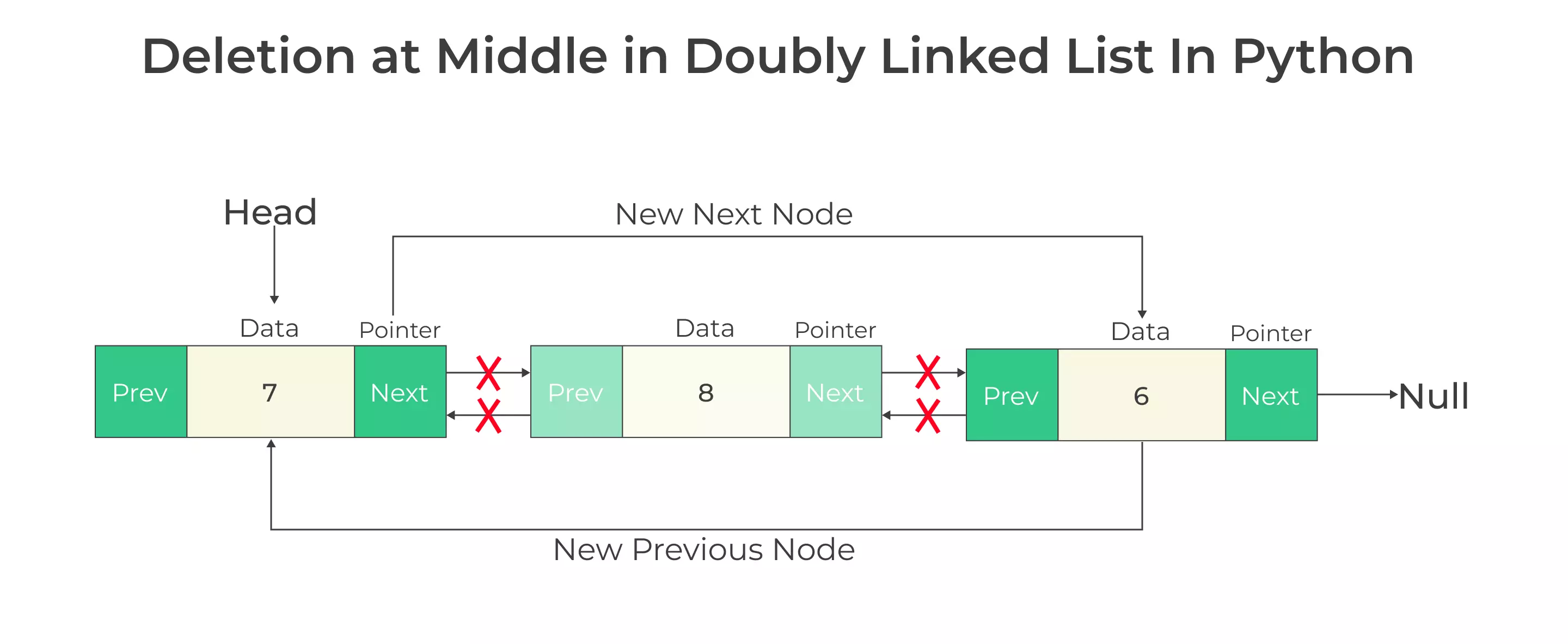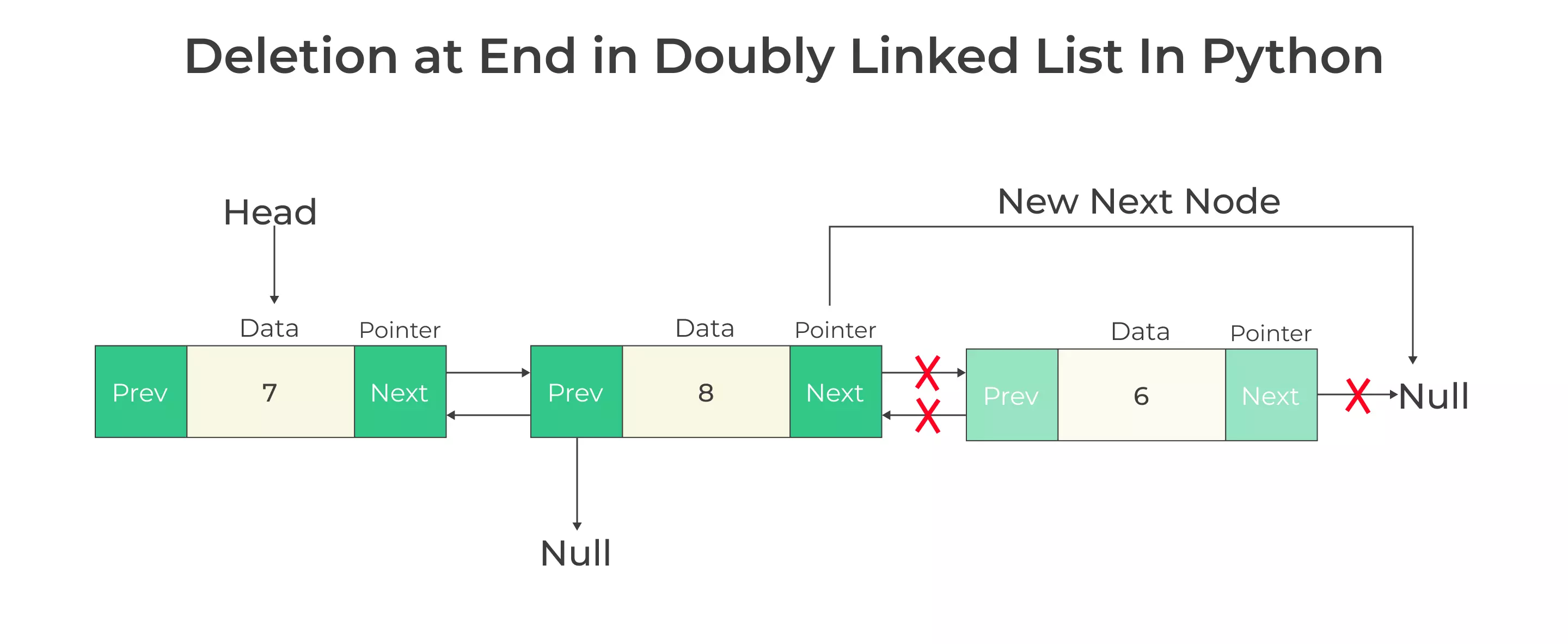# Deletion in Doubly Linked List in Python

## Deletion in Doubly Linked List in Python

Doubly linked lists are a fundamental data structure in computer science and are often used in various applications to efficiently manage and manipulate data.

In this page, we will explore how to perform deletion in doubly linked list using Python. We will cover different scenarios and techniques for deleting elements at various positions within the list## Understanding Deletion in Doubly Linked Lists in Python

A Doubly Linked List is a data structure that consists of a set of nodes, where each node contains both data and two pointers: one pointing to the next node and another pointing to the previous node. This bidirectional linking enables efficient traversal in both directions.

Deletion in Doubly linked list in Python involves removing a specific node from the list. Doubly linked lists consist of nodes, where each node contains two parts: data and a reference (or a pointer) to the next node in the list. To delete a node from a linked list, you typically need to update the references to ensure that the deleted node is no longer part of the list.

### Elements of Doubly Linked List

• Data : This is the actual information or value that the node holds. It can be of any data type, depending on the application’s requirements. For example, in a list of student records, the data may include the student’s name, ID, and grade.

• Next Pointer : The “next” pointer, also known as the “forward” or “next node” pointer, points to the next node in the list. It helps in navigating from the current node to the next node when traversing the list in the forward direction.

• Previous Pointer : The “previous” pointer, also known as the “backward” or “previous node” pointer, points to the previous node in the list. It facilitates traversal in the reverse direction, allowing you to move from the current node to the previous node.

### Deleting Nodes in Python Doubly Linked Lists

Deleting a node from a doubly linked list involves a few essential steps.

Step 1: Locate the Node to Delete

• Before deleting a node, we must find the node we want to remove. This typically involves traversing the list using a loop until we find the node with the desired data value.

Step 2: Update Pointers

• Once we’ve located the node to delete, we need to update the pointers of the adjacent nodes to bypass the node we’re deleting. This includes changing the ‘next’ pointer of the previous node to point to the next node and the ‘previous’ pointer of the next node to point to the previous node.

Step 3: Delete the Node

• After updating the pointers, we can safely delete the node from memory. In Python, memory management is typically automatic through garbage collection.

### Deletion in Doubly Linked-list in Python

For each deletion operation, we need to consider the three cases.

• Deletion at the beginning
• Deletion at Middle
• Deletion at End### Deletion at Beginning in Doubly Linked List in Python

#### Algorithm

• Check if there is only 1 node or not
• If there is one node
• Free memory
• Else
• Assign head to next node in the list
• Free memory

### Function for deletion at beginning in doubly linked list

```def delete_front(head):
# Check if the linked list is empty
print("Linked List Empty, nothing to delete")
return

# Check if Linked List has only 1 node
return

# Move head to the next node
# Assign the new head node's previous to None

print(f"{tempNode.data} deleted")
del tempNode
```

### Deletion at Middle in Doubly Linked List in Python

#### Algorithm

• Traverse till the target node
• create a node called the previous storing previous node of the target node
• Assign previous node’s next pointer to the next node of the target node
• For the next node of the target node, its previous pointer is assigned to the targets node’s previous node’s address
• Free memory of target node

### Function for deletion at Middle in doubly linked list

```class Node:
def __init__(self, data):
self.data = data
self.prev = None
self.next = None

def __init__(self):

def delete_nth_node(self, n):
print("Linked List Empty, nothing to delete")
return

len = self.get_length()

if n < 1 or n > len:
print("Enter valid position")
return

if n == 1:
self.delete_front()
return

if n == len:
self.delete_end()
return

# Traverse to the nth node
for i in range(n - 1):
temp_node = temp_node.next

previous_node = temp_node.prev  # (n-1)th node
next_node = temp_node.next  # (n+1)th node

# Assigning (n-1)th node's next pointer to (n+1)th node
previous_node.next = next_node

# Assigning (n+1)th node's previous pointer to (n-1)th node
next_node.prev = previous_node

# Deleting nth node
print(f"{temp_node.data} deleted")
del temp_node

def get_length(self):
length = 0
while temp_node:
length += 1
temp_node = temp_node.next
return length

def delete_front(self):
print("Linked List Empty, nothing to delete")
return

return

def delete_end(self):
print("Linked List Empty, nothing to delete")
return

return

while temp_node.next:
temp_node = temp_node.next

prev_node = temp_node.prev
prev_node.next = None

print(f"{temp_node.data} deleted")
del temp_node
```### Deletion at End in  Doubly Linked List in Python

• Traverse till the target node
• Check if this is the last node i.e. if node->next = NULL, then its last node
• Assign last node’s previous node’s next pointer to the last node’s next node’s address, which basically is NULL in this case
• Free the memory### Function for deletion at End in doubly linked list

```class Node:
def __init__(self, data):
self.data = data
self.prev = None
self.next = None

def __init__(self):

def delete_end(self):
print("Linked List Empty, nothing to delete")
return

if temp_node.next is None:
print(f"{temp_node.data} deleted")
return

# Traverse to the last node
while temp_node.next is not None:
temp_node = temp_node.next

second_last = temp_node.prev

# Assign 2nd last node's next to None
second_last.next = None

print(f"{temp_node.data} deleted")
del temp_node

# Usage example:
# Create a doubly linked list

# Insert some nodes (you can add more if needed)
node1 = Node(1)
node2 = Node(2)
node3 = Node(3)

node1.next = node2
node2.prev = node1
node2.next = node3
node3.prev = node2

# Delete the last node
dll.delete_end()
```

### Python Code for Deletion in a Doubly Linked List

Run
```class Node:
def __init__(self, data):
self.data = data
self.prev = None
self.next = None

def get_length(node):
length = 0
while node:
node = node.next
length += 1
return length

fresh_node = Node(data)

print("Linked List Empty, nothing to delete")
return None

return None

print("Linked List Empty, nothing to delete")
return None

return None

while temp_node.next:
temp_node = temp_node.next

second_last = temp_node.prev
second_last.next = None

print(f"{temp_node.data} deleted")

if n < 1 or n > length:
print("Enter valid position")

if n == 1:

if n == length:

while n > 1:
temp_node = temp_node.next
n -= 1

previous_node = temp_node.prev
next_node = temp_node.next

previous_node.next = next_node
next_node.prev = previous_node

print(f"{temp_node.data} deleted")

end = None

print("List in Forward direction:", end=" ")

print("\nList in backward direction:", end=" ")
while end:
print(end.data, end=" ")
end = end.prev

print("\n")

if __name__ == "__main__":

```

#### Output

```List in Forward direction:  12  11  10  9  8  7
List in backward direction: 7  8  9  10  11  12

12 deleted
List in Forward direction:  11  10  9  8  7
List in backward direction: 7  8  9  10  11

7 deleted
List in Forward direction:  11  10  9  8
List in backward direction: 8  9  10  11

9 deleted
List in Forward direction:  11  10  8
List in backward direction: 8  10  11

11 deleted
List in Forward direction:  10  8
List in backward direction: 8  10

8 deleted
List in Forward direction:  10
List in backward direction: 10
```

#### Time and Space Complexity

The time complexity of deletion in a Doubly Linked List is O(N), where N is the number of nodes. This is because, in the worst case, you may need to traverse the entire list to find the node to be deleted.

The space complexity is O(1), as no additional data structures are used for the deletion itself.

#### Conclusion

In this page, Deletion in Doubly Linked List in Python, we have discussed about the understanding the different scenarios for deletion and implementing them correctly is crucial for efficient data manipulation. Doubly linked lists provide an excellent foundation for various data-related tasks.

### Related Banners

Get PrepInsta Prime & get Access to all 200+ courses offered by PrepInsta in One SubscriptionQuestion 1.

What’s the process for deleting the tail node in a doubly linked list?

To delete the tail node, you update the previous pointer of the current tail and make the previous node the new tail.Question 2.

Can you delete a node in the middle of a doubly linked list?

Yes, you can delete a node in the middle by updating the next and previous pointers of the adjacent nodes to bridge the gap.Question 3.

How do I delete a node from a doubly linked list?

To delete a node, you can update the previous node’s next’ pointer to skip the node you want to delete and update the next node’s prev’ pointer accordingly.

## Get over 200+ course One Subscription

Courses like AI/ML, Cloud Computing, Ethical Hacking, C, C++, Java, Python, DSA (All Languages), Competitive Coding (All Languages), TCS, Infosys, Wipro, Amazon, DBMS, SQL and others